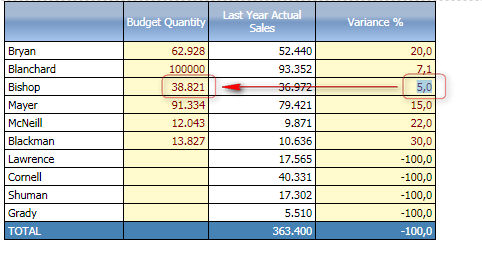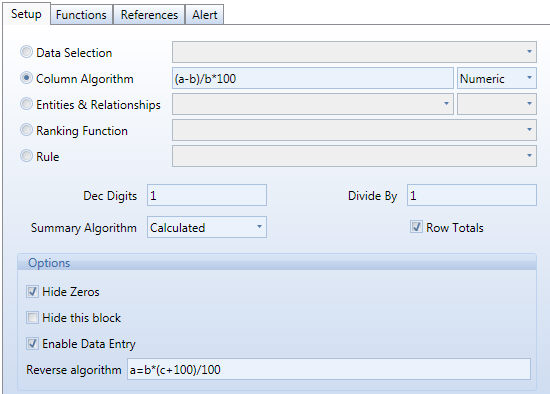﻿ Reverse algorithm

# Reverse algorithm

The reverse algorithm allows to key in a number on a calculated data block and save a value, derived through a reverse formula to a specified InfoCube.

## Example

Consider the following data-entry report:The data block (a) contains the Budget Quantity InfoCube and data entry has been enabled on this block. The user can enter a budget value on this block.

The second column, Last Year Actual Sales contains the sales value of last year, it is a reference value that helps the user defining the budget.

The third column, block (c), is the variance in percentage obtained with a column algorithm with the formula c=(a-b)/b*100. Data entry is enabled on this data block, allowing the user to key-in a percentage of variance and letting the system to derive the Budget Quantity corresponding to the given variance. The Budget quantity value is derived by applying the reverse formula a=b*(c+100)/100, this is the Reverse Algorithm.

In the reverse algorithm field, type the formula starting with the data block that is the variable to change when the user keys-in a value, followed by the equal sign and the formula itself as in the following example.Note:

The target data block of the reverse algorithm must be an InfoCube and must have the data entry option enabled.